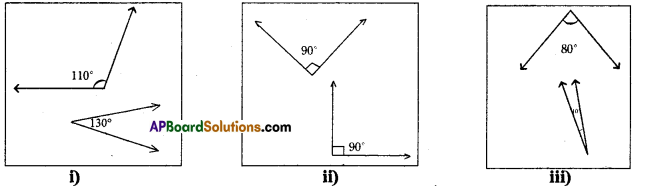# HSSlive: Plus One & Plus Two Notes & Solutions for Kerala State Board

## AP Board Class 7 Maths Chapter 4 Lines and Angles Ex 2 Textbook Solutions PDF: Download Andhra Pradesh Board STD 7th Maths Chapter 4 Lines and Angles Ex 2 Book AnswersAP Board Class 7 Maths Chapter 4 Lines and Angles Ex 2 Textbook Solutions PDF: Download Andhra Pradesh Board STD 7th Maths Chapter 4 Lines and Angles Ex 2 Book Answers

## Andhra Pradesh State Board Class 7th Maths Chapter 4 Lines and Angles Ex 2 Books Solutions

 Board AP Board Materials Textbook Solutions/Guide Format DOC/PDF Class 7th Subject Maths Chapters Maths Chapter 4 Lines and Angles Ex 2 Provider Hsslive

2. Click on the Andhra Pradesh Board Class 7th Maths Chapter 4 Lines and Angles Ex 2 Answers.
3. Look for your Andhra Pradesh Board STD 7th Maths Chapter 4 Lines and Angles Ex 2 Textbooks PDF.
4. Now download or read the Andhra Pradesh Board Class 7th Maths Chapter 4 Lines and Angles Ex 2 Textbook Solutions for PDF Free.

## AP Board Class 7th Maths Chapter 4 Lines and Angles Ex 2 Textbooks Solutions with Answer PDF Download

Find below the list of all AP Board Class 7th Maths Chapter 4 Lines and Angles Ex 2 Textbook Solutions for PDF’s for you to download and prepare for the upcoming exams:

Question 1.
Which of the following pairs of angles are complementary?Solution:
In fig (i) 110° – 30° = 140° ≠ 90. Hence they are not complementary.
In fig (ii) 90° + 90° = 180 ≠ 90°. Hence they are not complementary.
In fig (iii) 80° + 10° = 90° = 90. Hence they are complementary.

Question 2.
Find the complements of the given angles. Find the complementary angles of the following.
(i) 25°
(ii) 40°
(iii) 89°
(iv) 55°
Solution:
i) Complementary angle of 25° = 90° – 25° = 65°
ii) Complementary angle of 40° = 90° – 40° = 50°
ill) Complementary angle of 89° = 90° – 89° = 1°
iv) Complementary angle of 55° = 90° – 55 = 35°

Question 3.
Two angles are complement to each other and are also equal. Find them.
Solution:
Let the angle be x°
Then Its complement = (900_ x°)
By problem, x° = 90° – x°
x° + x°= 90°
2x = 90°
x = 90∘2
x = 45°

Question 4.
Manasa says, “Each angle in any pair of complementary angles is always acute”. Do
Solution:
Yes. Each angle ¡n any pair of complementary angles is always acute.
As sum of two angles is 90°, each of the angle must be less than 90°

## Andhra Pradesh Board Class 7th Maths Chapter 4 Lines and Angles Ex 2 Textbooks for Exam Preparations

Andhra Pradesh Board Class 7th Maths Chapter 4 Lines and Angles Ex 2 Textbook Solutions can be of great help in your Andhra Pradesh Board Class 7th Maths Chapter 4 Lines and Angles Ex 2 exam preparation. The AP Board STD 7th Maths Chapter 4 Lines and Angles Ex 2 Textbooks study material, used with the English medium textbooks, can help you complete the entire Class 7th Maths Chapter 4 Lines and Angles Ex 2 Books State Board syllabus with maximum efficiency.

## FAQs Regarding Andhra Pradesh Board Class 7th Maths Chapter 4 Lines and Angles Ex 2 Textbook Solutions

#### Can we get a Andhra Pradesh State Board Book PDF for all Classes?

Yes you can get Andhra Pradesh Board Text Book PDF for all classes using the links provided in the above article.

## Important Terms

Andhra Pradesh Board Class 7th Maths Chapter 4 Lines and Angles Ex 2, AP Board Class 7th Maths Chapter 4 Lines and Angles Ex 2 Textbooks, Andhra Pradesh State Board Class 7th Maths Chapter 4 Lines and Angles Ex 2, Andhra Pradesh State Board Class 7th Maths Chapter 4 Lines and Angles Ex 2 Textbook solutions, AP Board Class 7th Maths Chapter 4 Lines and Angles Ex 2 Textbooks Solutions, Andhra Pradesh Board STD 7th Maths Chapter 4 Lines and Angles Ex 2, AP Board STD 7th Maths Chapter 4 Lines and Angles Ex 2 Textbooks, Andhra Pradesh State Board STD 7th Maths Chapter 4 Lines and Angles Ex 2, Andhra Pradesh State Board STD 7th Maths Chapter 4 Lines and Angles Ex 2 Textbook solutions, AP Board STD 7th Maths Chapter 4 Lines and Angles Ex 2 Textbooks Solutions,
Share: